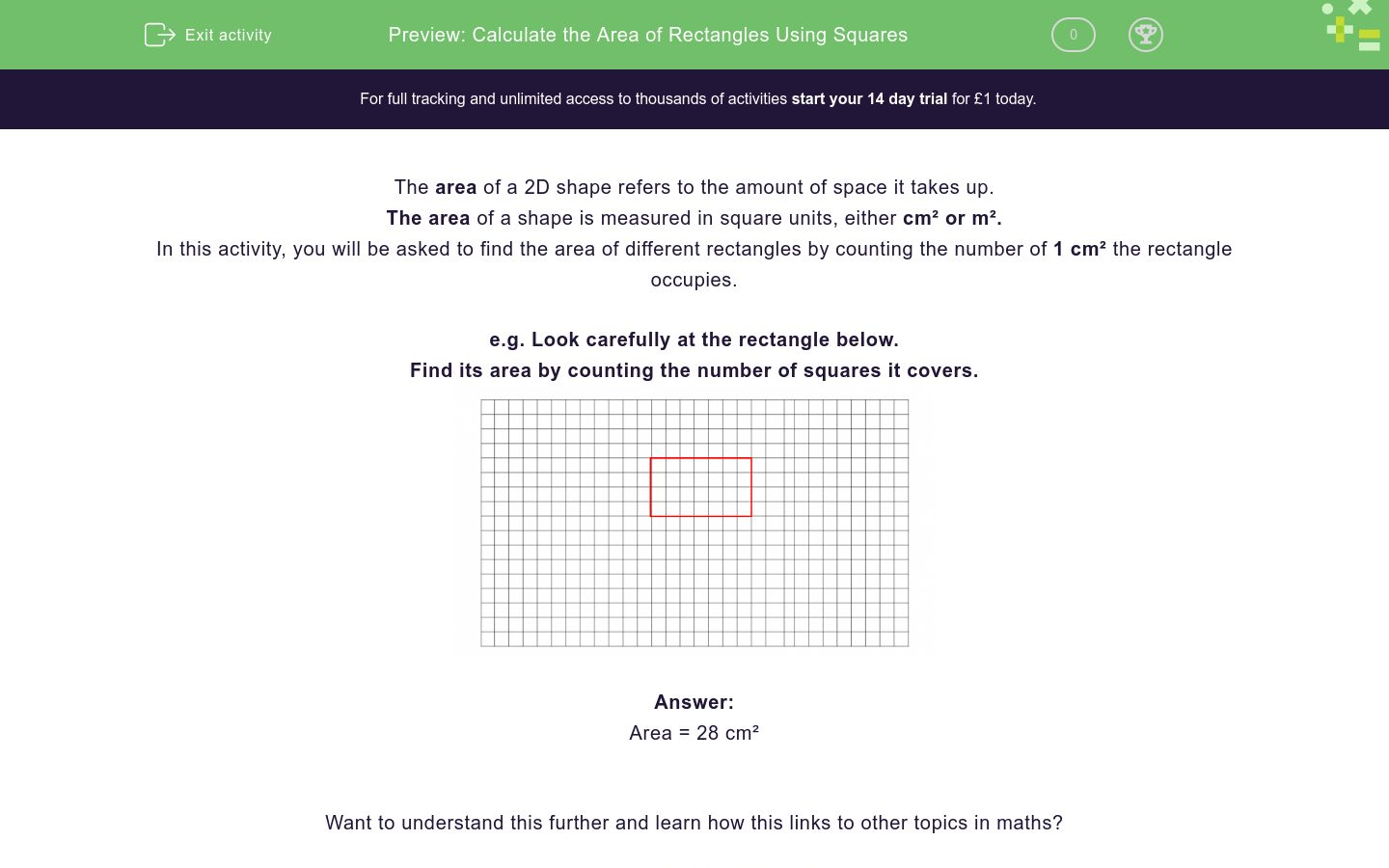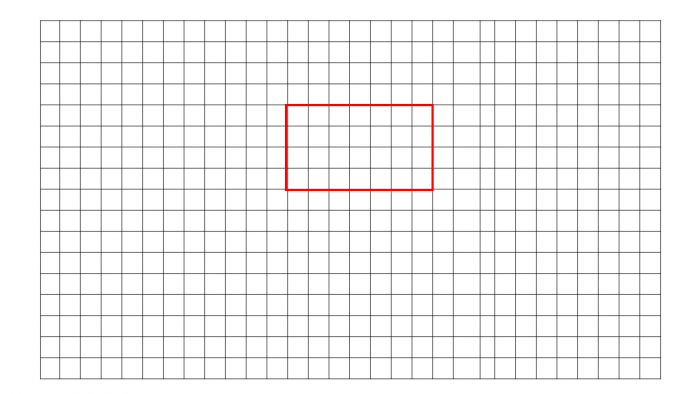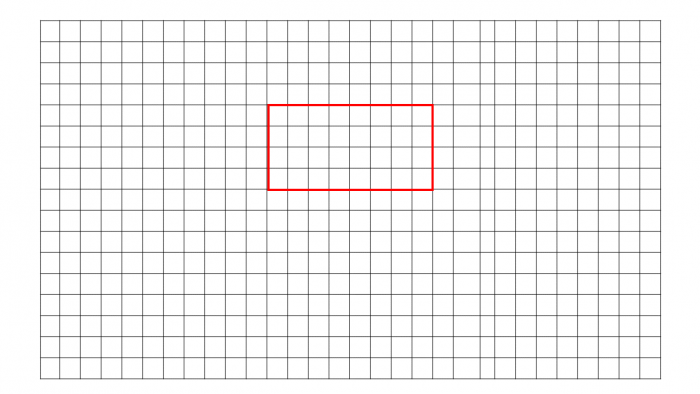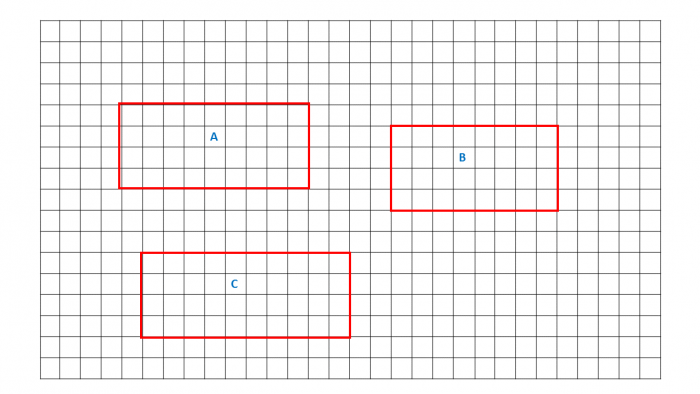# Calculate the Area of Rectangles Using Squares

In this worksheet, students will be asked to find the area of rectangles using squares and standard units, square centimetres and square metres.Key stage:  KS 2

Curriculum topic:   Measurement

Curriculum subtopic:   Calculate/Estimate Areas

Difficulty level:### QUESTION 1 of 10

The area of a 2D shape refers to the amount of space it takes up.

The area of a shape is measured in square units, either cm² or m².

In this activity, you will be asked to find the area of different rectangles by counting the number of 1 cm² the rectangle occupies.

e.g. Look carefully at the rectangle below.

Find its area by counting the number of squares it covers.Area = 28 cm²

Want to understand this further and learn how this links to other topics in maths?

Why not watch this video?

Find the area of the rectangle by counting the number of cm² covered.31 cm²

28 cm²

32 cm²

30 cm²

Find the area of the rectangle by counting the number of cm² covered.36 cm²

34 cm²

32 cm²

28 cm²

Find the area of the rectangle by counting the number of cm² covered.42 cm²

43 cm²

45 cm²

41 cm²

Find the area of the rectangle by counting the number of cm² covered.42 cm²

43 cm²

45 cm²

41 cm²

Find the area of the rectangle by counting the number of cm² covered.42 cm²

43 cm²

45 cm²

41 cm²

Find the area of the rectangle by counting the number of cm² covered.42 cm²

43 cm²

45 cm²

41 cm²

What is the area of this rectangle if each square is 1cm in length?20 cm² 16 cm² 22 cm² 18 cm² Area of Rectangle

Can you order the rectangles below from largest to smallest area by carefully counting the squares?## Column B

1st
C
2nd
A
3rd
B

Can you order the rectangles below from smallest to the largest area by carefully counting the squares?## Column B

1st
B
2nd
C
3rd
A

Read the following statements about rectangles carefully, and decide whether each is true or false.

## Column B

A square is also a rectangle
True
A rectangle is also a parallelogram
True
All rectangles contain two right angled triangles
False
A rectangle can also be called an oblong
Sometimes true
• Question 1

Find the area of the rectangle by counting the number of cm² covered.32 cm²
EDDIE SAYS
This rectangle has an area of 32 cm².
• Question 2

Find the area of the rectangle by counting the number of cm² covered.36 cm²
EDDIE SAYS
Count each square carefully, this rectangle has an area of 36 cm². This is a good start, don't worry if this is challenging at first, by the end of this worksheet you'll feel more confident.
• Question 3

Find the area of the rectangle by counting the number of cm² covered.45 cm²
EDDIE SAYS
I bet you're getting the hang of this now. This rectangle has an area of 45 cm².
• Question 4

Find the area of the rectangle by counting the number of cm² covered.EDDIE SAYS
This rectangle has an area of 50 cm².
• Question 5

Find the area of the rectangle by counting the number of cm² covered.EDDIE SAYS
How are you finding counting the squares to find the area? This rectangle has an area of 60 cm². You're now halfway through the worksheet...
• Question 6

Find the area of the rectangle by counting the number of cm² covered.EDDIE SAYS
This time the rectangle has an area of 66 cm².
• Question 7

What is the area of this rectangle if each square is 1cm in length?20 cm² 16 cm² 22 cm² 18 cm² Area of Rectangle
EDDIE SAYS
The area of the rectangle is 18 cm². Take a deep breath, you’ve got this.
• Question 8

Can you order the rectangles below from largest to smallest area by carefully counting the squares?## Column B

1st
C
2nd
A
3rd
B
EDDIE SAYS
We had to carefully count the squares for each rectangle in order to compare accurately. The order from largest to smallest area = C, A, B Area of the rectangles C = 40 cm² A = 36 cm² B = 32 cm²
• Question 9

Can you order the rectangles below from smallest to the largest area by carefully counting the squares?## Column B

1st
B
2nd
A
3rd
C
EDDIE SAYS
The question required that you carefully count the squares for each rectangle in order to compare accurately. The order from smallest to largest area B, A, C The area of the rectangles are; B = 40 cm² A = 44 cm² C = 48 cm²
• Question 10

Read the following statements about rectangles carefully, and decide whether each is true or false.

## Column B

A square is also a rectangle
True
A rectangle is also a parallelogr...
True
All rectangles contain two right ...
False
A rectangle can also be called an...
Sometimes true
EDDIE SAYS
This was a little challenge to finish the activity. 'A square is also a rectangle' is true. 'A rectangle is also a parallelogram' is true. 'All rectangles contain 2 right-angled triangles' is true. 'A rectangle can also be called an oblong' is sometimes true. Did that last one catch you out? Special well done if not! Practise makes perfect, that’s another activity ticked off.
---- OR ----

Sign up for a £1 trial so you can track and measure your child's progress on this activity.

### What is EdPlace?

We're your National Curriculum aligned online education content provider helping each child succeed in English, maths and science from year 1 to GCSE. With an EdPlace account you’ll be able to track and measure progress, helping each child achieve their best. We build confidence and attainment by personalising each child’s learning at a level that suits them.

Get started